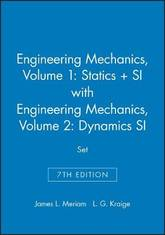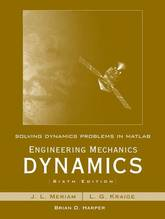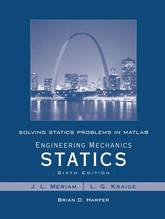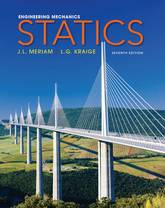#### L. G. Kraige author

Image credit: dr. shordzi, on Flickr
Filter
(found 40 products)SAVES YOUR STUDENT MONEY! SAVES YOUR STUDENTS MONEY!Provides a wide variety of high quality problems that are known for their accuracy, realism, applications, and variety. Students benefit from realistic applications that motivate their desire to learn and develop their problem solving skills. Sample Problems with a worked solution step appear ...
Engineering Mechanics: Statics: Volume 1: WITH Engineering Mechanics - Dynamics
SAVES YOUR STUDENT MONEY! SAVES YOUR STUDENTS MONEY!Provides a wide variety of high quality problems that are known for their accuracy, realism, applications, and variety. Students benefit from realistic applications that motivate their desire to learn and develop their problem solving skills. Sample Problems with a worked solution step appear throughout providing examples and reinforcing important concepts and idea in engineering mechanics Introductory Problems are simple, uncomplicated problems designed to help students gain confidence with a new topic. These appear in the problem sets following the Sample Problems. Representative Problems are more challenging than Introductory Problems but are of average difficulty and length. These appear in the problem sets following the Sample Problems. Computer-Oriented Problems are marked with an icon and appear in the end-of-chapter Review Problems. Review Problems appear at the end of chapter.Offers comprehensive coverage of how to draw free body diagrams. Through text discussion and assignable homework problems students will learn that drawing free body diagrams is the most important skill needed to learn how to solve mechanics problems. Meriam and Kraige teach students the appropriate techniques and then apply them consistently in solutions of mechanics problems.SI Units are covered. There are approximately two problems in SI units for every one in U.S. customary units.A tradition of excellence. Since 1952 this text has been a primary source for accuracy, rigor, clarity and a high standard of illustration in the coverage of mechanics theory.
116.90 USD

## Engineering Mechanics: Statics: Volume 1: WITH Engineering Mechanics - Dynamics

by L. G. Kraige, James L Meriam
Paperback / softbackAn introduction to MATLAB for engineering students, complete with practice problems Written as a complement to Engineering Mechanics Dynamics, this book provides students with an introduction to MATLAB as well as example problems that correspond to the aforementioned text. The book covers numerical calculations, defining functions, graphics, symbolic calculations, differentiation ...
Solving Dynamics Problems in MATLAB to accompany Engineering Mechanics Dynamics 6e
An introduction to MATLAB for engineering students, complete with practice problems Written as a complement to Engineering Mechanics Dynamics, this book provides students with an introduction to MATLAB as well as example problems that correspond to the aforementioned text. The book covers numerical calculations, defining functions, graphics, symbolic calculations, differentiation and integration, and solving equations with MATLAB and then presents problems in seven subsequent chapters. These cover kinematics of particles, kinetics of particles, kinetics of systems of particles; plane kinematics of rigid bodies; plane kinetics of rigid bodies, three-dimensional dynamics of rigid bodies, and vibration and response time.
55.600000 USD

## Solving Dynamics Problems in MATLAB to accompany Engineering Mechanics Dynamics 6e

by L. G. Kraige, J. L. Meriam, Brian Harper
Paperback / softbackLearn MATLAB to help you understand mechanics MATLAB is a powerful tool for mathematical modeling, and is becoming essential to many engineering fields. Solving Statics Problems in MATLAB is designed to both introduce students to MATLAB, and provide them with a useful tool for understanding static mechanics. The book begins ...
Solving Statics Problems in MATLAB to accompany Engineering Mechanics Statics 6e
Learn MATLAB to help you understand mechanics MATLAB is a powerful tool for mathematical modeling, and is becoming essential to many engineering fields. Solving Statics Problems in MATLAB is designed to both introduce students to MATLAB, and provide them with a useful tool for understanding static mechanics. The book begins with MATLAB basics, including scripts, functions, graphics, calculations, and equations, then moves into physics with targeted guidance on solving force systems, equilibriums, structures, friction, and more. The problems presented are taken from Engineering Mechanics Statics, 6th Edition, but the clear guidance and comprehensive instruction can be applied to any course.
56.650000 USD

## Solving Statics Problems in MATLAB to accompany Engineering Mechanics Statics 6e

by L. G. Kraige, J. L. Meriam, Brian Harper
Paperback / softbackIn the latest edition of Engineering Mechanics, Meriam and Kraige deliver a uniquely powerful learning solution: a sound conceptual foundation for solving mechanics problems that is the hallmark of the Meriam text. The new edition offers easy-to-use tools and resources to drive positive outcomes/success. Most notably, it retains the hallmark ...
Engineering Mechanics: Statics
In the latest edition of Engineering Mechanics, Meriam and Kraige deliver a uniquely powerful learning solution: a sound conceptual foundation for solving mechanics problems that is the hallmark of the Meriam text. The new edition offers easy-to-use tools and resources to drive positive outcomes/success. Most notably, it retains the hallmark of the Meriam texts by teaching realistic engineering problem solving without sacrificing rigor. Other features include easy-to-use assessment tools to create effective learning pathways through the text, immediate feedback and remediation, along with problem solving practice to help develop confidence and take control. The text seamlessly integrates additional examples and practice exercises that are crafted to bridge the gap between a conceptual grasp from lectures and the textbook to the solution of homework and exam problems. Presentation resources, automated scoring, diagnostic reporting and tools enable consistent delivery.